# Prime numbers

(notes by Roberto Bigoni)

## 1. Prime numbers

As we know from our early arithmetic studies, prime numbers are the natural numbers greater than 1, which aren't the product of two other numbers both less than the number itself.

This definition implies that 1 is not a prime number, and that the only even prime number is 2.

Prime numbers are infinite, that is, the set of prime numbers does not have maximum. A proof by contradiction of this theorem is due to Euclid.

If prime numbers were not infinite, then would exist the maximum prime number M, and it would be possible to calculate the number P = 2·3·5·7·11·13·…·M, equal to the product of all the prime numbers.

Let us consider the natural number S immediately following P: S=P+1.

It is impossible that S is a prime number, because it is greater than the maximum M.

If we were to divide S for any prime number, we would have a quotient given by the product of the remaining prime numbers and the remainder 1, then S is not divisible by any of the prime numbers, so it must be prime.

We get two contradictory statements and we must therefore conclude that the primes are infinite.

Natural numbers greater than 1 that are not prime are said composite numbers.

## 2. The Sieve of Eratosthenes

The easiest method to get the set Pn of the primes ≤ n (n>2) is the Sieve of Eratosthenes
(sieve: a tool used for separating coarse from fine parts of loose matter):

1. we initialize the set Pn containing the number 2;
2. we consider each of the following numbers k, 2 < kn;
if k is not divisible by a number pPn, k is added to the set Pn;
3. at the end of the procedure, Pn contains all the prime numbers pn.

To determine whether an odd number n is prime, ie to check its primality, we may construct the set Pn and check whether n is the last element of Pn.

The following Javascript application calculates the prime numbers ≤ n and the n-th prime number.
Obviously, it functions properly only if your browser has JavaScript enabled.
If your browser does not allow internal frames, you can directly access the application page.
If the calculation time exceeds the written minutes, you can try to increase them.

## 3. Fermat's factorization method.

But if n is very large, the sieve becomes impractical because it may require too much memory and too much computation time. Primality tests that do not require the previous calculation of a huge number of primes, were searched and are searched even today.

One of such tests may be derived from a factorization method due to P. de Fermat.

Given the odd number n, the Fermat's factorization method is based on the research of two numbers h and k such thatIf n is a perfect square, the solution is immediate because in this case n is equal to the product of its square root for itself and, of course, n is not prime.

If n is not too big it is convenient to apply the Sieve of Eratosthenes.

Otherwise we can always express hk as a difference of two squares: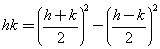then, with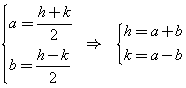we get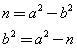The problem is solved if we find a number a such that a2-n is a perfect square.

For this purpose we proceed by trials:

• we assume as the first value of a the first natural number greater than its square root;
• if a2-n is a perfect square, the problem is solved;
• otherwise we increase a by 1 until a2-n is a perfect square;
• at this point we calculate the square root a2-n getting b.

Given a e b, we have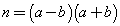If a-b = 1, n is prime; otherwise the two factors a-b and a-b (that is h e k) are different and less than n, then n is composite.

## 4. Perfect squares

Fermat's factorization method requires that we repeatedly check whether a natural number n is a perfect square. It is not possible to directly determine this property of n. However, in many cases we can eliminate quickly enough this possibility, noting that residues modulo m, for a given square number m, are quite a few.

For example, the quadratic residues modulo 8 for the squares of the first 100 natural numbers, using WolframAlpha, areEven without a formal proof, it can safely be conjectured that by calculating the remainder of the division of a natural number by 8, if the remainder is equal to 0, 1, 4, the number is not a perfect square.

A further example is provided by the set of the quadratic residues modulo 9.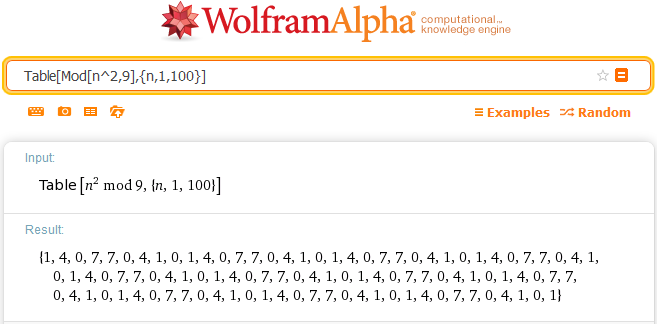Even in this case we can safely conjecture that by calculating the remainder of the division of a number by 9, if the remainder is equal to 0, 1, 4, 7, the number is not a perfect square.

But to pass a test of this kind is necessary but not sufficient condition to conclude that n is a perfect square. It is a useful control to narrow down the search for possible perfect squares, but not conclusive. To successfully identify a perfect square we inevitably need a more expensive procedure, like the following bisection algorithm.

1. We consider the sequence of the natural numbers qi that, starting from 4, is formed by numbers such that each of them is the square of the previous number.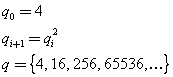2. If n coincides with one of these numbers, n is a perfect square, and the procedure ends.
3. Otherwise even its square root √n does not coincide with a qi and then it is comprised between two of these terms:;
4. we let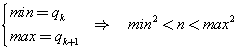5. we compare n with the square of the mean value m: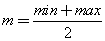• If n=m2, n is a perfect square, its square root is m and the procedure ends.
• If max ≤ min+1, n is not a perfect square, and the procedure ends.
• n < m2 we set max = m, otherwise we set min = m and we return to the step 5.

Example.

Let n = 40401

We have 40401≡1 (mod 8) and 40401≡0 (mod 9), so n passes the two proposed preliminary tests. Now we apply the bisection algorithm:

 step 1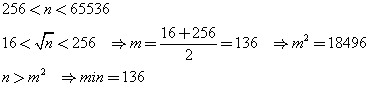step 2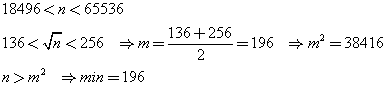step 3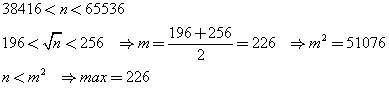step 4step 5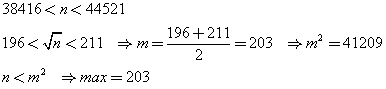step 6step 7endn is a perfect square; its square root is 201

## 5. The Fermat primality test

A more direct method is even due to Fermat and is based on a theorem enunciated by Fermat himself, but subsequently demonstrated by L. Euler, known as Fermat's little theorem.

If p is a prime number, then, for any integer n,where npn(mod p) means that the divisions by p of np and n give equal remainders. We say that np and n are congruent modulo p.

For example: given n=10 and p=3, we have:

• 103=1000
• 1000:3=333 with remainder 1
• 10:3=3 with remainder 1

A demonstration of the theorem may be obtained by induction.

First of all we observe that, if p is prime,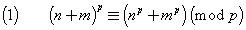In fact, if we expand the power of the binomial, we get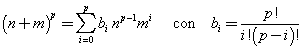where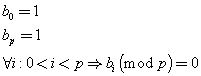The theorem holds for n=0 and n=1: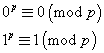If the theorem holds for n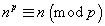from the equation (1) we get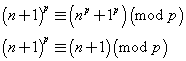Then, by induction, the theorem holds for all n.

In particular, if n has no common divisors with p, that is it is coprime with respect to p, we have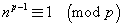The theorem states a necessary, but not sufficient condition, that is any prime p verify the stated congruence, but this congruence may be verified also by some composite number c. These numbers are said Fermat pseudoprimes with respect to n. In particular, the pseudoprimes c with respect to any n coprime with respect to c are said Carmichael numbers.

So, if a number q does not verify the theorem with respect to a coprime n, we can say that q is composite, but if it verifies the congruence, we can not say that it is prime, but only that it is a probable prime.

If we try with many random values of n with always positive results, we can operationally assume q as a prime number.

Examples.

1. Let q=41.

• With n=2, we have 240=1099511627776, 240≡1 (mod 41). 41 pass the test: it is a probable prime.
• With n=3, we have 340=12157665459056928801, 340≡1 (mod 41). 41 pass also this test: it is a probable prime with greater probability.
• With n=5, we have 540=9094947017729282379150390625, 540≡1 (mod 41). 41 pass also this test: it is a probable prime with even greater probability.

The probability that q is not a prime number is quite small. Indeed 41 is prime, as we can check with the Sieve of Eratosthenes.

2. Let q=91.

• With n=2, we have 290=1237940039285380274899124224, 240≡64 (mod 91). 91 does not pass the test: it is composite. Indeed 91=7*13

3. Let q=561.

If we apply the Fermat's factorization method, we get 561 = 17 · 33. So 561 is composite.

Now we try the Fermat primality test:

• Let n=2,
we have 2560=37739624248215413522415545809882688909169212204164404283762063002456241623921488...
52086126725177658767541468375030763844899770584629924792632561434251432696043649395326976.
2560≡1 (mod 561).
561, although it isn't prime, passes the test, and it would pass it for any other n coprime with respect to 561. Indeed 561 is the smallest number of Carmichael.

## 6. Miller-Rabin primality test

The Fermat primality test, even with several trials, can fail, that is it can lead us to consider as prime a number that instead is composite. We can reduce this risk and estimate the probability of getting the correct answer, noting that a prime number q>2 must be odd and then q-1 must be even. Moreover, every even number can be decomposed into the product of an odd number d and a power s of 2.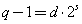By Fermat's theorem, if q is prime, and n is coprime with respect to q,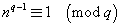, we have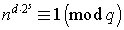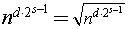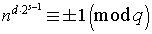If this root is ≡ 1(mod q), then the next will be ≡ ±1 (mod q), and so on.

If all the roots are ≡ 1 (mod q), so even nd≡1 (mod q), q passes the test and is probably prime.

If the first root not ≡ 1 (mod q) is = -1 (mod q), q passes the test and is probably prime.

Otherwise q is composite.

In conclusion, to determine whether q is probably prime:

• we randomly choose a number n coprime with respect to q;
• if, with this choice, q does not pass the Fermat test, q is composite;
• else
• we calculate nd (mod q); if we get 1, the number is probably prime;
• otherwise, we calculate the powersmodulo q with k decreasing from s-1 to 0. If the first of these powers not ≡ 1 (mod q) is not ≡ -1 (mod q), q is composite;
• otherwise q passes the test and is probably prime.

The probability that a composite number q passes the test is at most ¼. Therefore, by repeating the test with other values of n, the probability that q can pass them all decreases exponentially.

Examples.

1. As we have seen the number q=561, is a Fermat pseudoprime, therefore, while being composite, it passes the Fermat test.

We decompose 560 into the product between an odd number and a power of 2: 560=35·24

With n=2, we have 235=34359738368; 235≡ 263 (mod 561)

35·23=280;
2280=1942668892225729070919461906823518906642406839052139521251812409738904285205208498176
2280≡1 (mod 561)

35·22=140;
2140=1393796574908163946345982392040522594123776
2140≡67 (mod 561)

So q is composite.

2. Let q=601

With n=2, we have
2600=414951556888099295851240786369116115101244623224243689999565732969065281141290814639970704894710379428
8197886611300789182395151075411775307886874834113963687061181803401509523685376
2600≡1 (mod 601): the number passes the Fermat test.

600=75*23

With n=2 we have

275=37778931862957161709568; 275≡ 1 (mod 601): 601 is a probable prime.

3. Let q=401

With n=2, we have
2400=2582249878086908589655919172003011874329705792829223512830659356540647622016841194629645353280137831435903171972747493376
2400≡1 (mod 401): 401 passes the Fermat test.

400=25·24

With n=2 we have

225=33554432; 275≡356 (mod 401)

2200=1606938044258990275541962092341162602522202993782792835301376; 2200≡ 1 (mod 401);

2100=1267650600228229401496703205376; 2100≡ -1 (mod 401): 401 is a probable prime.

## 7. Prime factorization of a number

A natural number n, greater than 1, either it is prime or it is composite.

Using a reliable test like that of Miller-Rabin, one can directly decide whether it is prime and therefore whether its factors are only 1 and n.

If n is composite we can use the sieve of Eratosthenes.

1. We calculate the set Pn = {p1, p2, … pn } of the prime numbers less than the square root of n.
2. If n is divisible by a pi, pi, is a factor of the decomposition; we divide and check whether the quotient is still divisible by pi.
If yes, the divisions by pi are repeated until a non-divisible quotient is obtained. pi appears in the factorization with exponent equal to the number of divisions made.
3. The procedure is reapplied to the last quotient obtained by considering the remaining elements of Pn.

However, if n is very big, even √n is big and so is the number of the elements of Pn, the calculation of which may require impractical time.

If we try the Fermat method and the method is successful, n can be expressed as the product of two natural numbers h and k less than n. In their turn, each of the numbers h and k either is prime or is composite. If they are both prime, their product is the decomposition of n into prime factors product (or factorization of n). Otherwise, we can apply the Fermat's method to the composite factors and repeat the process until we have only prime factors. If at the end of the procedure a factor appears several times, the product of the identical factors is replaced by a power and the product of these powers (with exponent ≥ 1) is the unique decomposition of n into product of powers of prime factors.

The Fermat's method works satisfactorily for not too big numbers or, even for big numbers, if the two factors h and k are both next to √n. Otherwise, it may take an impractical computing time even on the fastest systems.

To overcome these difficulties, other methods of factoring have been proposed, such as, for example, the following algorithm due to John M. Pollard.

## 8. Pollard's ρ (rho) algorithm

Let h be a divisor of n.

If, given s0 = a and the function f(s) = mod(s2+2,n), we construct the sequence S where si+1 = f( si ), then, after at most h+1 steps, we find a ss such that ss = sk (k > s) and then the subsequent elements repeat cyclically.

Representing the elements of S as nodes of a graph, this takes on a shape that recalls the Greek letter ρ (Latin transcription 'rho', corresponding to our r lowercase, hence the name of the algorithm).

For example, given n = 51, h = 3 is a divisor of n. If we let s0 = 2, we have

isif( si )
026
1638
23818
31820
42045
54538
63818
71820
82045
94538

s2 = s6, then s3 = s7 and so on.

If ij, sj > si e sjsi (mod h), we have:

• si = x h + a
• sj = y h + a
• sj - si = ( y - x ) h

therefore

GCD ( sj - si , n ) = h

If, continuing the proposed example, we construct a table of the absolute values of the differences di,j = si - sj between all the calculated sk, we observe that, ij, only in some cases the GCD(di,j,n) is greater than 1 and that in these cases GCD = 3, ie h.

 2 6 38 18 20 45 38 18 20 45 2 0 4 36 16 18 43 36 16 18 43 6 4 0 30 12 14 39 30 12 14 39 38 36 32 0 20 18 7 0 20 18 7 18 16 12 20 0 2 27 20 0 2 27 20 18 14 18 2 0 25 18 2 0 25 45 43 39 7 27 25 0 7 27 25 0 38 36 30 0 20 18 7 0 20 18 7 18 16 12 20 0 2 27 20 0 2 27 20 18 14 18 2 0 25 18 2 0 25 45 43 39 7 27 25 0 7 27 25 0

So if we choose randomly si and sj with ij and GCD(di,j,n) > 1, then GCD (di,j,n) = h.

We can avoid random attempts by matching the terms of the sequence S with those of the sequence T in which, given t0 = 2, ti+1 = f( f( ti ) ).

In the proposed example:

isiti| ti - si |
0220
163832
2382018
3183820
420200
545387
6382018
7183820
820200
945387

Obviously the set of terms of the sequence T is a subset of the set of terms of S, so the difference between a term of T and a term of S is also a difference between two terms of S. By matching the terms of the two sequences and calculating the GCD between n and the absolute value of the difference ti - si, if the GCD is greater than 1, it is a divisor of n ie h.

We can then calculate k = n / h.

Now

1. n is expressed as the product of two natural numbers h and k less than n;
2. in turn, h and k are prime or composite;
3. if they are both prime, their product is the decomposition of n into a prime factors product;
4. otherwise it is possible to apply the sieve or Fermat's method or the Pollard's algorithm to the composite factors and repeat the procedure until only prime factors are obtained;
5. if, at the end of the procedure, a factor appears several times, the product of the identical factors is replaced by a power and the product of these powers (with exponent ≥) is the decomposition of n product of powers of prime factors.

## 9. Calculator

The following Javascript application performs the above described calculations.
Obviously, it functions properly only if your browser has JavaScript enabled.
If your browser does not allow internal frames, you can directly access the application page.
If the calculation time exceeds the written minutes, you can try to increase them.

## 10. Very big numbers.

The applications of this page are implemented in JS and, while enhanced by the BigInt library of Leemon Baird, they are inevitably too slow to handle very big numbers. If we have to decompose very big numbers, we can try to use WolframAlpha.

It should however be noted that, even using the most advanced software and specially designed hardware, the factorization of a very big number may require unworkable computational time and this fact is the basis of the most efficient encryption methods, such as RSA.

last revision: December 2018